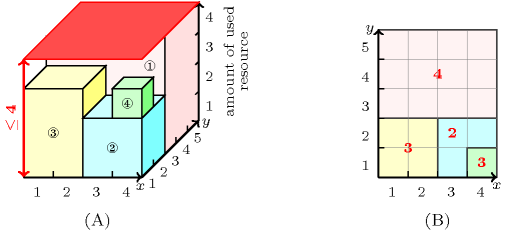## 5.99. cumulative_two_d

Origin
Constraint

$\mathrm{𝚌𝚞𝚖𝚞𝚕𝚊𝚝𝚒𝚟𝚎}_\mathrm{𝚝𝚠𝚘}_𝚍\left(\mathrm{𝚁𝙴𝙲𝚃𝙰𝙽𝙶𝙻𝙴𝚂},\mathrm{𝙻𝙸𝙼𝙸𝚃}\right)$

Arguments
 $\mathrm{𝚁𝙴𝙲𝚃𝙰𝙽𝙶𝙻𝙴𝚂}$ $\mathrm{𝚌𝚘𝚕𝚕𝚎𝚌𝚝𝚒𝚘𝚗}\left(\begin{array}{c}\mathrm{𝚜𝚝𝚊𝚛𝚝}\mathtt{1}-\mathrm{𝚍𝚟𝚊𝚛},\hfill \\ \mathrm{𝚜𝚒𝚣𝚎}\mathtt{1}-\mathrm{𝚍𝚟𝚊𝚛},\hfill \\ \mathrm{𝚕𝚊𝚜𝚝}\mathtt{1}-\mathrm{𝚍𝚟𝚊𝚛},\hfill \\ \mathrm{𝚜𝚝𝚊𝚛𝚝}\mathtt{2}-\mathrm{𝚍𝚟𝚊𝚛},\hfill \\ \mathrm{𝚜𝚒𝚣𝚎}\mathtt{2}-\mathrm{𝚍𝚟𝚊𝚛},\hfill \\ \mathrm{𝚕𝚊𝚜𝚝}\mathtt{2}-\mathrm{𝚍𝚟𝚊𝚛},\hfill \\ \mathrm{𝚑𝚎𝚒𝚐𝚑𝚝}-\mathrm{𝚍𝚟𝚊𝚛}\hfill \end{array}\right)$ $\mathrm{𝙻𝙸𝙼𝙸𝚃}$ $\mathrm{𝚒𝚗𝚝}$
Restrictions
 $\mathrm{𝚛𝚎𝚚𝚞𝚒𝚛𝚎}_\mathrm{𝚊𝚝}_\mathrm{𝚕𝚎𝚊𝚜𝚝}$$\left(2,\mathrm{𝚁𝙴𝙲𝚃𝙰𝙽𝙶𝙻𝙴𝚂},\left[\mathrm{𝚜𝚝𝚊𝚛𝚝}\mathtt{1},\mathrm{𝚜𝚒𝚣𝚎}\mathtt{1},\mathrm{𝚕𝚊𝚜𝚝}\mathtt{1}\right]\right)$ $\mathrm{𝚛𝚎𝚚𝚞𝚒𝚛𝚎}_\mathrm{𝚊𝚝}_\mathrm{𝚕𝚎𝚊𝚜𝚝}$$\left(2,\mathrm{𝚁𝙴𝙲𝚃𝙰𝙽𝙶𝙻𝙴𝚂},\left[\mathrm{𝚜𝚝𝚊𝚛𝚝}\mathtt{2},\mathrm{𝚜𝚒𝚣𝚎}\mathtt{2},\mathrm{𝚕𝚊𝚜𝚝}\mathtt{2}\right]\right)$ $\mathrm{𝚛𝚎𝚚𝚞𝚒𝚛𝚎𝚍}$$\left(\mathrm{𝚁𝙴𝙲𝚃𝙰𝙽𝙶𝙻𝙴𝚂},\mathrm{𝚑𝚎𝚒𝚐𝚑𝚝}\right)$ $\mathrm{𝚁𝙴𝙲𝚃𝙰𝙽𝙶𝙻𝙴𝚂}.\mathrm{𝚜𝚒𝚣𝚎}\mathtt{1}\ge 0$ $\mathrm{𝚁𝙴𝙲𝚃𝙰𝙽𝙶𝙻𝙴𝚂}.\mathrm{𝚜𝚒𝚣𝚎}\mathtt{2}\ge 0$ $\mathrm{𝚁𝙴𝙲𝚃𝙰𝙽𝙶𝙻𝙴𝚂}.\mathrm{𝚑𝚎𝚒𝚐𝚑𝚝}\ge 0$ $\mathrm{𝙻𝙸𝙼𝙸𝚃}\ge 0$
Purpose

Consider a set $ℛ$ of rectangles described by the $\mathrm{𝚁𝙴𝙲𝚃𝙰𝙽𝙶𝙻𝙴𝚂}$ collection. Enforces that at each point of the plane, the cumulated height of the set of rectangles that overlap that point, does not exceed a given limit.

Example
$\left(\begin{array}{c}〈\begin{array}{ccccccc}\mathrm{𝚜𝚝𝚊𝚛𝚝}\mathtt{1}-1\hfill & \mathrm{𝚜𝚒𝚣𝚎}\mathtt{1}-4\hfill & \mathrm{𝚕𝚊𝚜𝚝}\mathtt{1}-4\hfill & \mathrm{𝚜𝚝𝚊𝚛𝚝}\mathtt{2}-3\hfill & \mathrm{𝚜𝚒𝚣𝚎}\mathtt{2}-3\hfill & \mathrm{𝚕𝚊𝚜𝚝}\mathtt{2}-5\hfill & \mathrm{𝚑𝚎𝚒𝚐𝚑𝚝}-4,\hfill \\ \mathrm{𝚜𝚝𝚊𝚛𝚝}\mathtt{1}-3\hfill & \mathrm{𝚜𝚒𝚣𝚎}\mathtt{1}-2\hfill & \mathrm{𝚕𝚊𝚜𝚝}\mathtt{1}-4\hfill & \mathrm{𝚜𝚝𝚊𝚛𝚝}\mathtt{2}-1\hfill & \mathrm{𝚜𝚒𝚣𝚎}\mathtt{2}-2\hfill & \mathrm{𝚕𝚊𝚜𝚝}\mathtt{2}-2\hfill & \mathrm{𝚑𝚎𝚒𝚐𝚑𝚝}-2,\hfill \\ \mathrm{𝚜𝚝𝚊𝚛𝚝}\mathtt{1}-1\hfill & \mathrm{𝚜𝚒𝚣𝚎}\mathtt{1}-2\hfill & \mathrm{𝚕𝚊𝚜𝚝}\mathtt{1}-2\hfill & \mathrm{𝚜𝚝𝚊𝚛𝚝}\mathtt{2}-1\hfill & \mathrm{𝚜𝚒𝚣𝚎}\mathtt{2}-2\hfill & \mathrm{𝚕𝚊𝚜𝚝}\mathtt{2}-2\hfill & \mathrm{𝚑𝚎𝚒𝚐𝚑𝚝}-3,\hfill \\ \mathrm{𝚜𝚝𝚊𝚛𝚝}\mathtt{1}-4\hfill & \mathrm{𝚜𝚒𝚣𝚎}\mathtt{1}-1\hfill & \mathrm{𝚕𝚊𝚜𝚝}\mathtt{1}-4\hfill & \mathrm{𝚜𝚝𝚊𝚛𝚝}\mathtt{2}-1\hfill & \mathrm{𝚜𝚒𝚣𝚎}\mathtt{2}-1\hfill & \mathrm{𝚕𝚊𝚜𝚝}\mathtt{2}-1\hfill & \mathrm{𝚑𝚎𝚒𝚐𝚑𝚝}-1\hfill \end{array}〉,4\hfill \end{array}\right)$

Part (A) of Figure 5.99.1 shows the 4 parallelepipeds of height 4, 2, 3 and 1 associated with the items of the $\mathrm{𝚁𝙴𝙲𝚃𝙰𝙽𝙶𝙻𝙴𝚂}$ collection (parallelepipeds since each rectangle also has a height). Part (B) gives the corresponding cumulated 2-dimensional profile, where each number is the cumulated height of all the rectangles that contain the corresponding region. The $\mathrm{𝚌𝚞𝚖𝚞𝚕𝚊𝚝𝚒𝚟𝚎}_\mathrm{𝚝𝚠𝚘}_𝚍$ constraint holds since the highest peak of the cumulated 2-dimensional profile does not exceed the upper limit 4 imposed by the last argument of the $\mathrm{𝚌𝚞𝚖𝚞𝚕𝚊𝚝𝚒𝚟𝚎}_\mathrm{𝚝𝚠𝚘}_𝚍$ constraint.

##### Figure 5.99.1. Two representations of a 2-dimensional cumulative profile of the Example slot (where the profile provides for each point of coordinates $\left({c}_{x},{c}_{y}\right)$ the corresponding sum of the heights of the items intersecting that point): (A) a three dimensional representation and (B) a two dimensional representation from above with the height of the profile in red; as for the $\mathrm{𝚌𝚞𝚖𝚞𝚕𝚊𝚝𝚒𝚟𝚎}$ constraint the position of an item on the $z$ axis does not matter, i.e. only its height matters.Typical
 $|\mathrm{𝚁𝙴𝙲𝚃𝙰𝙽𝙶𝙻𝙴𝚂}|>1$ $\mathrm{𝚁𝙴𝙲𝚃𝙰𝙽𝙶𝙻𝙴𝚂}.\mathrm{𝚜𝚒𝚣𝚎}\mathtt{1}>0$ $\mathrm{𝚁𝙴𝙲𝚃𝙰𝙽𝙶𝙻𝙴𝚂}.\mathrm{𝚜𝚒𝚣𝚎}\mathtt{2}>0$ $\mathrm{𝚁𝙴𝙲𝚃𝙰𝙽𝙶𝙻𝙴𝚂}.\mathrm{𝚑𝚎𝚒𝚐𝚑𝚝}>0$ $\mathrm{𝙻𝙸𝙼𝙸𝚃}<$$\mathrm{𝚜𝚞𝚖}$$\left(\mathrm{𝚁𝙴𝙲𝚃𝙰𝙽𝙶𝙻𝙴𝚂}.\mathrm{𝚑𝚎𝚒𝚐𝚑𝚝}\right)$
Symmetries
• Items of $\mathrm{𝚁𝙴𝙲𝚃𝙰𝙽𝙶𝙻𝙴𝚂}$ are permutable.

• Attributes of $\mathrm{𝚁𝙴𝙲𝚃𝙰𝙽𝙶𝙻𝙴𝚂}$ are permutable w.r.t. permutation $\left(\mathrm{𝚜𝚝𝚊𝚛𝚝}\mathtt{1},\mathrm{𝚜𝚝𝚊𝚛𝚝}\mathtt{2}\right)$ $\left(\mathrm{𝚜𝚒𝚣𝚎}\mathtt{1},\mathrm{𝚜𝚒𝚣𝚎}\mathtt{2}\right)$ $\left(\mathrm{𝚕𝚊𝚜𝚝}\mathtt{1},\mathrm{𝚕𝚊𝚜𝚝}\mathtt{2}\right)$ $\left(\mathrm{𝚑𝚎𝚒𝚐𝚑𝚝}\right)$ (permutation applied to all items).

• $\mathrm{𝚁𝙴𝙲𝚃𝙰𝙽𝙶𝙻𝙴𝚂}.\mathrm{𝚑𝚎𝚒𝚐𝚑𝚝}$ can be decreased to any value $\ge 0$.

• One and the same constant can be added to the $\mathrm{𝚜𝚝𝚊𝚛𝚝}\mathtt{1}$ and $\mathrm{𝚕𝚊𝚜𝚝}\mathtt{1}$ attributes of all items of $\mathrm{𝚁𝙴𝙲𝚃𝙰𝙽𝙶𝙻𝙴𝚂}$.

• One and the same constant can be added to the $\mathrm{𝚜𝚝𝚊𝚛𝚝}\mathtt{2}$ and $\mathrm{𝚕𝚊𝚜𝚝}\mathtt{2}$ attributes of all items of $\mathrm{𝚁𝙴𝙲𝚃𝙰𝙽𝙶𝙻𝙴𝚂}$.

• $\mathrm{𝙻𝙸𝙼𝙸𝚃}$ can be increased.

Arg. properties

Contractible wrt. $\mathrm{𝚁𝙴𝙲𝚃𝙰𝙽𝙶𝙻𝙴𝚂}$.

Usage

The $\mathrm{𝚌𝚞𝚖𝚞𝚕𝚊𝚝𝚒𝚟𝚎}_\mathrm{𝚝𝚠𝚘}_𝚍$ constraint is a necessary condition for the $\mathrm{𝚍𝚒𝚏𝚏𝚗}$ constraint in 3 dimensions (i.e., the placement of parallelepipeds in such a way that they do not pairwise overlap and that each parallelepiped has his sides parallel to the sides of the placement space).

Algorithm

A first natural way to handle this constraint would be to accumulate the compulsory part [Lahrichi82] of the different rectangles in a quadtree [Samet89]. To each leave of the quadtree we associate the cumulated height of the rectangles containing the corresponding region.

Systems

geost in Choco.

related: $\mathrm{𝚍𝚒𝚏𝚏𝚗}$ ($\mathrm{𝚌𝚞𝚖𝚞𝚕𝚊𝚝𝚒𝚟𝚎}_\mathrm{𝚝𝚠𝚘}_𝚍$ is a necessary condition for $\mathrm{𝚍𝚒𝚏𝚏𝚗}$: forget one dimension when the number of dimensions is equal to 3).
specialisation: $\mathrm{𝚋𝚒𝚗}_\mathrm{𝚙𝚊𝚌𝚔𝚒𝚗𝚐}$ ($\mathrm{𝚜𝚚𝚞𝚊𝚛𝚎}$ of size 1 with a $\mathrm{𝚑𝚎𝚒𝚐𝚑𝚝}$ replaced by $\mathrm{𝚝𝚊𝚜𝚔}$ of $\mathrm{𝚍𝚞𝚛𝚊𝚝𝚒𝚘𝚗}$ 1), $\mathrm{𝚌𝚞𝚖𝚞𝚕𝚊𝚝𝚒𝚟𝚎}$ ($\mathrm{𝚛𝚎𝚌𝚝𝚊𝚗𝚐𝚕𝚎}$ with a $\mathrm{𝚑𝚎𝚒𝚐𝚑𝚝}$ replaced by $\mathrm{𝚝𝚊𝚜𝚔}$ with same $\mathrm{𝚑𝚎𝚒𝚐𝚑𝚝}$).